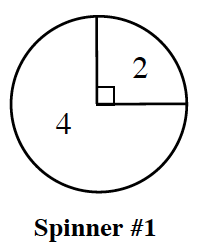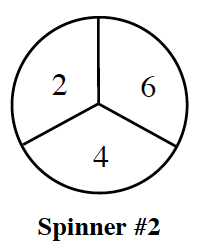### Home > INT2 > Chapter 3 > Lesson 3.1.4 > Problem3-42

3-42.

The spinners below are spun and the results are added. Assume each of the outcomes on Spinner #2 are equally likely.1. What is $P$(sum is $4$)?

Draw an area model or tree diagram.

2. What is $P$(sum is $8$)?

Using the area model or tree diagram you made in part (a), circle all the places on your model where the sum is $8$.

$P(\text{sum is 8})=\frac{1}{12}+\frac{1}{4}=\frac{1}{3}$